##### Statistics For Dummies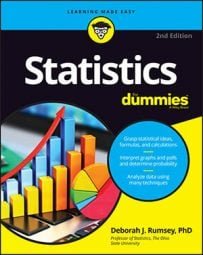Can one statistic measure both the strength and direction of a linear relationship between two variables? Sure! Statisticians use the correlation coefficient to measure the strength and direction of the linear relationship between two numerical variables X and Y. The correlation coefficient for a sample of data is denoted by r.

Although the street definition of correlation applies to any two items that are related (such as gender and political affiliation), statisticians use this term only in the context of two numerical variables. The formal term for correlation is the correlation coefficient. Many different correlation measures have been created; the one used in this case is called the Pearson correlation coefficient.

The formula for the correlation (r) is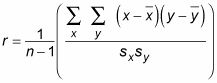where n is the number of pairs of data;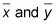are the sample means of all the x-values and all the y-values, respectively; and sx and sy are the sample standard deviations of all the x- and y-values, respectively.

You can use the following steps to calculate the correlation, r, from a data set:

1. Find the mean of all the x-values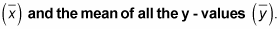2. Find the standard deviation of all the x-values (call it sx) and the standard deviation of all the y-values (call it sy).

For example, to find sx, you would use the following equation: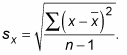3. For each of the n pairs (x, y) in the data set, take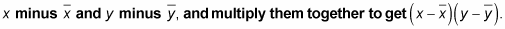4. Add up the n results from Step 3.

5. Divide the sum by sxsy.

6. Divide the result by n – 1, where n is the number of (x, y) pairs. (It’s the same as multiplying by 1 over n – 1.)

This gives you the correlation, r.

For example, suppose you have the data set (3, 2), (3, 3), and (6, 4). You calculate the correlation coefficient r via the following steps. (Note that for this data the x-values are 3, 3, 6, and the y-values are 2, 3, 4.)
1. Calculating the mean of the x and y values, you get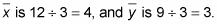2. The standard deviations are sx = 1.73 and sy = 1.00.

3. The n = 3 differences found in Step 2 multiplied together are: (3 – 4)(2 – 3) = (– 1)( – 1) = +1; (3 – 4)(3 – 3) = (– 1)(0) = 0; (6 – 4)(4 – 3) = (2)(1) = +2.

4. Adding the n = 3 Step 3 results, you get 1 + 0 + 2 = 3.

5. Dividing by sxsy gives you 3 / (1.73 ∗ 1.00) = 3 / 1.73 = 1.73. (It’s just a coincidence that the result from Step 5 is also 1.73.)

6. Now divide the Step 5 result by 3 – 1 (which is 2), and you get the correlation r = 0.87.